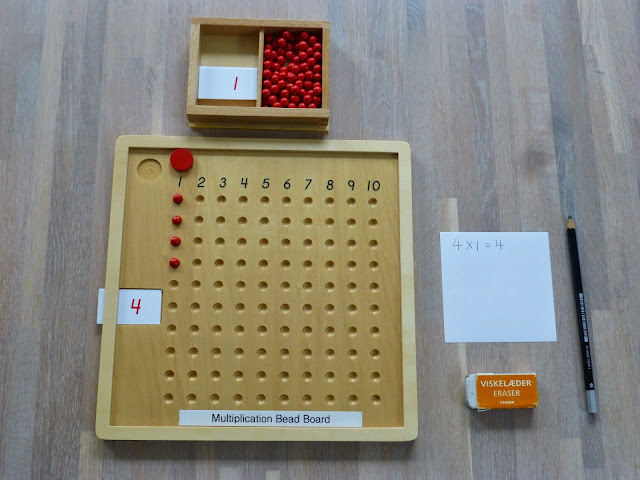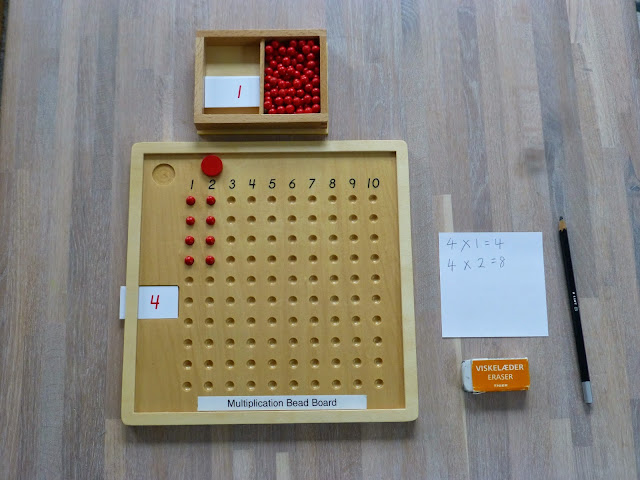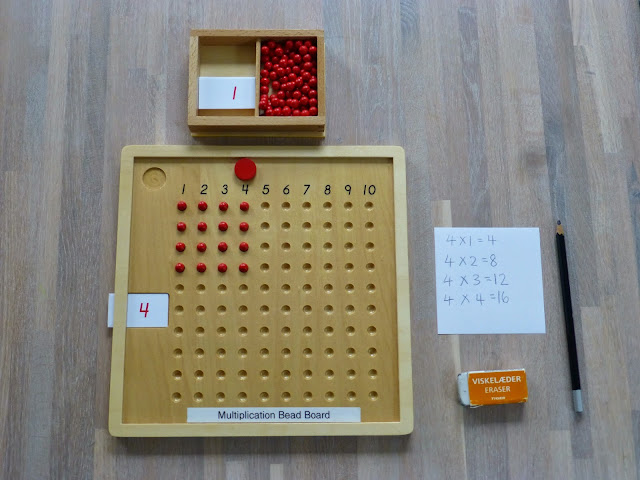## Wednesday, 21 January 2015

### Montessori Activity: Multiplication Bead Board (乘法珠板 [chéngfǎ zhū bǎn])The Multiplication Bead Board

AGE: From 6 years old (after your child who has worked with the bead bar layout to do this exercise)

OBJECTIVE(S):

1. To teach the concept of multiplicand and multiplier in a visual and concrete manner.
2. To allow practice aiding in the memorization of the multiplication tables.

DIFINITION OF MULTIPLICATION:

Explain that:
- Multiplication (乘法) is adding the same number over and over again, e.g. 3+3 means you have 3 two times, and we write it as 3x2; 3+3+3 means you have 3 three times, and we write it as 3x3; and 3+3+3+3 means you have 3 four times, we write it as 3x4.

- Multiplicand (被乘数[bèi chéng shù]) is the number that gets multiplied, in this case, it is 3.

- Multiplier (乘数 [chéng shù]) is the number that you are multiplying by, in this case, it is 2,3 and 4.

MATERIALS:
1. 1 Multiplication Bead Board (On multiplication board slot on left is for insertion of card indicating the number of the set with which child is working. Numbers printed across the top of the board indicate number of sets which have been placed on the board.)

2. 1 multiplication worksheet, 1 pencil and eraser.

3. 1 mat

PRESENTATION:
1. Set up the materials as shown and tell your child that we will be working on multiplication. This example will be made with Time Table 4.

﻿Multiplicand 4 with multiplier 1
2. Write out the problem e.g. 4x1=? and read it together with your child.(四乘以一得多少?)

3. Place 4 beads in the column directly below 1 and say, "4 shown 1 time is 4", counting as you go along, thus forming 1 set of 4 or 4x1=4 (四乘以一得四).

4. Place number card 4 into the slot which indicates the multiplicand.

5. Place a red disc in the indent directly above the printed 1 at the top left of the board indicating the multiplier 1.

6. Explain that 4 (multiplicand) appearing one time (multiplier) will give 4.

7. Explain to your child that the numbers along the top are the multiplier i.e. the number of times the multiplicand are repeated.

9. Write out the second problem e.g. 4x2=? and have your child read it.Multiplicand 4 with multiplier 2
10. Place the next set of 4 beads in the column directly below 2, counting as you go along from the previous set 4, 5, 6, 7 and 8, saying, "2 set of 4 or 4x2=8 (四乘以二得八).

11. Say, “We already have 4 one time, and we now have 4 two times. 4x2 gives you 8.”

12. Then move the disc in the indent directly above the printed 2 at the top left of the board indicating the multiplier 2.

13. Record the answer on the worksheet.

14. Invite your child to try and continue in same way to 10 sets of 4 or 4x10=40.Multiplicand 4 with multiplier 3Multiplicand 4 with mulptiplier 4

VIDEO DEMONSTRATION:

More video demonstrations here:

CONTROL OF ERROR:

2. Control chart 1.

Our Little FECS (5Y10M16D) tried this for the first time today 21 Jan 2015. We tried 2 time table to 6 time table. He was able to understand the concept, and also pointed out the pattern in multiplication such as 2, 4, 6, 8, 10. This is one of the Montessori materials that I love, as it really makes the concept of multiplication very concrete to children. He finds the 5 time table the easiest, as it is simplying just interchanging the numbers with 5 and 10.

REFERENCES:

Brilliant Minds Montessori Maths Curriculum

The Multiplication Bead Board is available from Amazon:21 Jan 2015 (5Y10M16D)22 Jan 2015 (5Y10M17D) - J completed the 6 time-table after we came back from the Fun City and music class, while waiting for me to make pancakes. He was able to complete it on his own, adding up all the 6s along the way.